# 2 Covariant Formalism

According to the pattern of classical hydrodynamics, we decompose the spacetime metric into the spatial metric and the instantaneous rest-space of a comoving observer. The formalism, known as the 3 + 1 covariant approach to general relativity,[25,26,27,28,29,30] has been used for numerous applications.[10,31,32,33,34,35] In this approach, we rewrite equations governing relativistic fluid dynamics by using projected vectors and projected symmetric traceless tensors instead of metrics.[10,34]

We take a four-velocity vectorfield in a given (3 + 1)-dimensional spacetime to be a unit vector field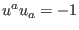. We define a spatial metric (or projector tensor)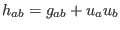, whereis the spacetime metric. It decomposes the spacetime metric into the spatial metric and the instantaneous rest-space of an observer moving with four-velocity.[2,33,36] We get some properties for the spatial metric(1)

We also define the spatial alternating tensor as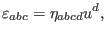(2)

whereis the spacetime alternating tensor,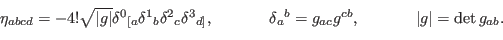(3)

The covariant spacetime derivative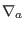is split into a covariant temporal derivative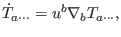(4)

and a covariant spatial derivative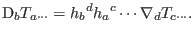(5)

The projected vectors and the projected symmetric traceless parts of rank-2 tensors are defined by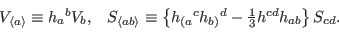(6)

The equations governing these quantities involve a vector product and its generalization to rank-2 tensors: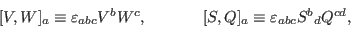(7)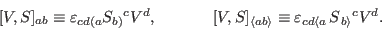(8)

We define divergences and rotations as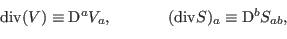(9)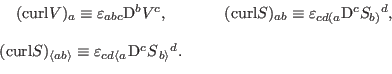(10)

We know that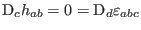,, and, then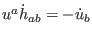and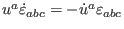. From these points one can also define the relativistically temporal rotations as(11)

The covariant spatial distortions are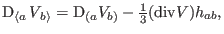(12)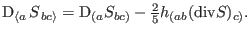(13)

We decompose the covariant derivatives of scalars, vectors, and rank-2 tensors into irreducible components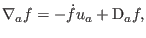(14)(15)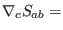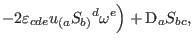(16)

where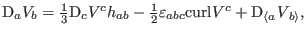(17)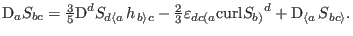(18)

We also introduce the kinematic quantities encoding the relative motion of fluids: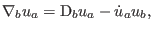(19)(20)

where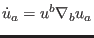is the relativistic acceleration vector, in the frames of instantaneously comoving observers,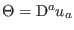the rate of expansion of fluids,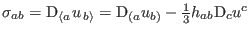a traceless symmetric tensor (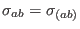,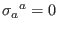); the shear tensor describing the rate of distortion of fluids, and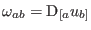a skew-symmetric tensor (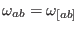,); the vorticity tensor describing the rotation of fluids.[27,33,37]

The vorticity vector[38,39]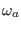is defined by(21)

where,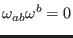and the magnitudehave been imposed. Accordingly, we obtain(22)

The sign convention is such that in the Newtonian theory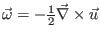.

We denote the covariant shear and vorticity products of the symmetric traceless tensors as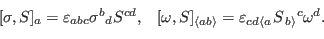(23)

The energy density and pressure of fluids are encoded in the dynamic quantities, which generally have the contributions from the energy flux and anisotropic pressure: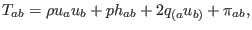(24)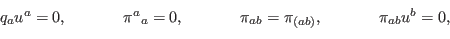(25)

where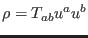is the relativistic energy density relative to,the pressure,the energy flux relative to, andthe traceless anisotropic stress. Imposing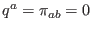, we get the solution of a perfect fluid with. In addition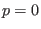gives the pressure-free matter or dust solution.[27,33,37]

Ashkbiz Danehkar
2018-03-26